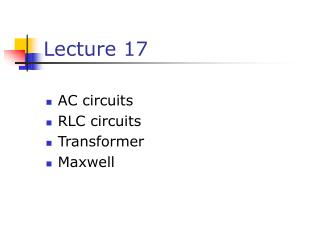Download PresentationLecture 17

# Lecture 17 - PowerPoint PPT PresentationDownload Presentation## Lecture 17

- - - - - - - - - - - - - - - - - - - - - - - - - - - E N D - - - - - - - - - - - - - - - - - - - - - - - - - - -
##### Presentation Transcript

1. Lecture 17 • AC circuits • RLC circuits • Transformer • Maxwell

2. AC Circuit • An AC circuit consists of a combination of circuit elements and an AC generator or source • The output of an AC generator is sinusoidal and varies with time according to the following equation • Δv = ΔVmax sin 2ƒt • Δv is the instantaneous voltage • ΔVmax is the maximum voltage of the generator • ƒ is the frequency at which the voltage changes, in Hz

3. Resistor in an AC Circuit • Consider a circuit consisting of an AC source and a resistor • The graph shows the current through and the voltage across the resistor • The current and the voltage reach their maximum values at the same time • The current and the voltage are said to be in phase Demo

4. More About Resistors in an AC Circuit • The direction of the current has no effect on the behavior of the resistor • The rate at which electrical energy is dissipated in the circuit is given by • where i is the instantaneous current • the heating effect produced by an AC current with a maximum value of Imax is not the same as that of a DC current of the same value • The maximum current occurs for a small amount of time

5. rms Current and Voltage • The rms current is the direct current that would dissipate the same amount of energy in a resistor as is actually dissipated by the AC current • Alternating voltages can also be discussed in terms of rms values

6. Power Revisited • The average power dissipated in resistor in an AC circuit carrying a current I is

7. Table 21-1, p.695

8. Fig. 21-1, p.694

9. Ohm’s Law in an AC Circuit • rms values will be used when discussing AC currents and voltages • AC ammeters and voltmeters are designed to read rms values • Many of the equations will be in the same form as in DC circuits • Ohm’s Law for a resistor, R, in an AC circuit • ΔVR,rms = Irms R • Also applies to the maximum values of v and i

10. Capacitors in an AC Circuit • Consider a circuit containing a capacitor and an AC source • The current starts out at a large value and charges the plates of the capacitor • There is initially no resistance to hinder the flow of the current while the plates are not charged • As the charge on the plates increases, the voltage across the plates increases and the current flowing in the circuit decreases

11. More About Capacitors in an AC Circuit • The current reverses direction • The voltage across the plates decreases as the plates lose the charge they had accumulated • The voltage across the capacitor lags behind the current by 90° Demo

12. Capacitive Reactance and Ohm’s Law • The impeding effect of a capacitor on the current in an AC circuit is called the capacitive reactance and is given by • When ƒ is in Hz and C is in F, XC will be in ohms • Ohm’s Law for a capacitor in an AC circuit • ΔVC,rms = Irms XC

13. Inductors in an AC Circuit • Consider an AC circuit with a source and an inductor • The current in the circuit is impeded by the back emf of the inductor • The voltage across the inductor always leads the current by 90° Demo

14. Inductive Reactance and Ohm’s Law • The effective resistance of a coil in an AC circuit is called its inductive reactance and is given by • XL = 2ƒL • When ƒ is in Hz and L is in H, XL will be in ohms • Ohm’s Law for the inductor • ΔVL,rms = Irms XL

15. The RLC Series Circuit • The resistor, inductor, and capacitor can be combined in a circuit • The current in the circuit is the same at any time and varies sinusoidally with time

16. Current and Voltage Relationships in an RLC Circuit • The instantaneous voltage across the resistor is in phase with the current • The instantaneous voltage across the inductor leads the current by 90° • The instantaneous voltage across the capacitor lags the current by 90°

17. Fig. 21-8, p.699